Courses

# Test: Flow Over Notches & Weirs

## 10 Questions MCQ Test Topicwise Question Bank for Mechanical Engineering | Test: Flow Over Notches & Weirs

Description
This mock test of Test: Flow Over Notches & Weirs for Mechanical Engineering helps you for every Mechanical Engineering entrance exam. This contains 10 Multiple Choice Questions for Mechanical Engineering Test: Flow Over Notches & Weirs (mcq) to study with solutions a complete question bank. The solved questions answers in this Test: Flow Over Notches & Weirs quiz give you a good mix of easy questions and tough questions. Mechanical Engineering students definitely take this Test: Flow Over Notches & Weirs exercise for a better result in the exam. You can find other Test: Flow Over Notches & Weirs extra questions, long questions & short questions for Mechanical Engineering on EduRev as well by searching above.
QUESTION: 1

Solution:
QUESTION: 2

### The discharge of broad crested weir is maximum if the head of water on the downstream side of weir as compared to the head on the upstream side of the weir is

Solution: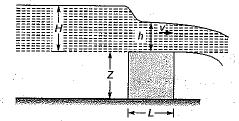If 2L > H, the weir is called broad-crested weir.
If 2 L < H, the weir is called narrow -crested weir by Bernoulli's equation to the still water surface on the upstream side and running water at the end of weir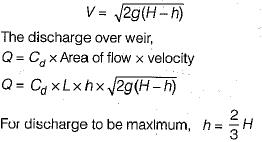QUESTION: 3

### Maximum discharge over broad crested weir is

Solution: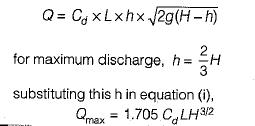QUESTION: 4

The cipoletti weir functions as if it were a following notch without end contractions

Solution: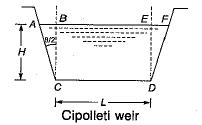Cipolieti weir is a trapezoidal weir which has side slopes of 1 horizontal to 4 vertical as shown.
By giving slope to the sides, an increase in discharge through the triangular portions ABC and DEFof the weir would be rectangular.

QUESTION: 5

Cipolleti notch is designed as trapezoid with sides sloping at 1 horizontal and

Solution:
QUESTION: 6

What is the discharge over a cipolleti weir of length 2 m when the head over the weir is 1 m? (Take Cd = 0.62)

Solution: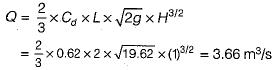QUESTION: 7

Match List - I ( Discharge  of ) with List-11 (Proportional to)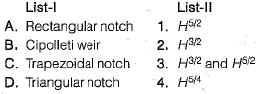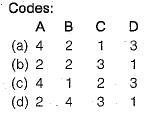Solution:
QUESTION: 8

The approximate discharge over a 4 m long rectangular suppresed weir with head over the crest as 0.36 m is

Solution: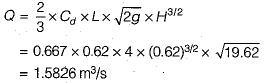QUESTION: 9

The discharge over a suppresed sharp crested rectangular weir is given by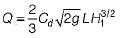Here H1 is the difference in elevation-between

Solution:
QUESTION: 10

For a suppressed rectangular weir an arrangement for aeration of nappe is necessary to

Solution: